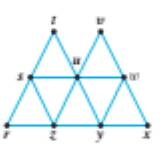Chapter 10.1, Problem 15ES### Discrete Mathematics With Applicat...

5th Edition
EPP + 1 other
ISBN: 9781337694193

#### Solutions

Chapter
Section### Discrete Mathematics With Applicat...

5th Edition
EPP + 1 other
ISBN: 9781337694193
Textbook Problem
1 views

# Determine which of the graph in 12-17 have Euler circuits. If the graph does not have an Euler circuit, explain why not. If it does have an Euler circuit, describe one.To determine

Determine which graph have Euler circuits. If the graph does not have an Euler circuit, explain why not. If it does have an Euler circuit, describe one.

Explanation

Given information:

Calculation:

The graph has an Euler circuit because it is connected and every vertex has a positive even degree...

### Still sussing out bartleby?

Check out a sample textbook solution.

See a sample solution

#### The Solution to Your Study Problems

Bartleby provides explanations to thousands of textbook problems written by our experts, many with advanced degrees!

Get Started